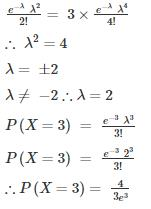Courses

# Engineering Mathematics 8

## 10 Questions MCQ Test RRB JE for Computer Science Engineering | Engineering Mathematics 8

Description
This mock test of Engineering Mathematics 8 for Computer Science Engineering (CSE) helps you for every Computer Science Engineering (CSE) entrance exam. This contains 10 Multiple Choice Questions for Computer Science Engineering (CSE) Engineering Mathematics 8 (mcq) to study with solutions a complete question bank. The solved questions answers in this Engineering Mathematics 8 quiz give you a good mix of easy questions and tough questions. Computer Science Engineering (CSE) students definitely take this Engineering Mathematics 8 exercise for a better result in the exam. You can find other Engineering Mathematics 8 extra questions, long questions & short questions for Computer Science Engineering (CSE) on EduRev as well by searching above.
QUESTION: 1

### Given  X ¬ B (n,p) if E(X) = 6, Var(X) = 4.2 then what is the value of n?

Solution: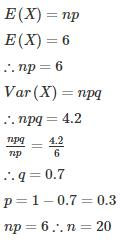QUESTION: 2

### The probability density function of the normal distribution of a random variable X is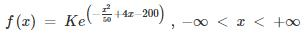What is the sum of mean and standard deviation?

Solution: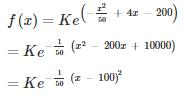Probability density function of normal distribution is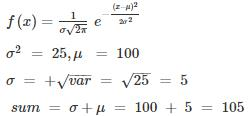Note:
σ = standard deviation

σ= variance

μ = mean

QUESTION: 3

### Binomial distribution B(n,p) can be approximated to a normal distribution N(np, np(1−p)) if ____

Solution:

Binomial distribution B(n,p) can be approximated to normal distribution N(np,np(1−p)) if n is large and p and 1-p are almost equal. Approximation generally improves as n increases (at least 20) and is better when p is not near to 0 or 1.

*Answer can only contain numeric values
QUESTION: 4

If a random variable x satisfies the Poisson’s distribution with a mean value of 3, then the probability that (x ≥ 2) is Poisson’s distribution,

Solution:

For Poisson’s distribution,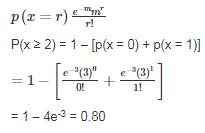QUESTION: 5

Let Harsh and Dinesh be the two players playing chess and their chances of winning a game are in the ration 4:3 respectively. What is the chance of Dinesh winning at least 4 games out of five games played?

Solution: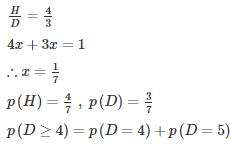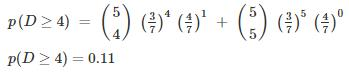*Answer can only contain numeric values
QUESTION: 6

From an urn containing 3 red and 2 white balls, a man is to draw 2 balls at random without replacement, being promised Rs. 20 for each red ball he draws and Rs. 10 for each white one. Find his expectation (In rupees).

Solution:

A man is to draw 2 balls at random without replacement.

Probability to draw 2 red balls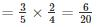Probability to draw 2 white balls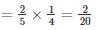Probability to draw one red ball and one white ball =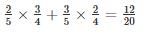Let X be the random variable and it shows the money he earn.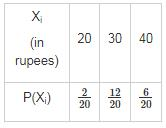Expectation =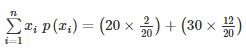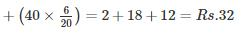*Answer can only contain numeric values
QUESTION: 7

If the probability of passing an exam is 0.001, then determine the chance that more than 3 students out of 3,000 will pass the exam (answer up to 2 decimal place)?

Solution:

Poisson distribution is used as probability of occurrence is very small.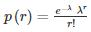mean = λ = n

∴ λ = 3000 × 0.001 = 3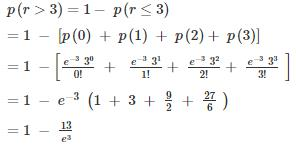= 0.35

QUESTION: 8

A dice is rolled 180180 times, find the probability that face 44 will turn up at least 3535 times.(Assume normal distribution). Assume p(0 < z < 1) = 0.3413

Solution:

Given that n = 180

Required Probability is given by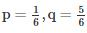∴ mean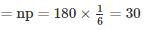Variance = npq = 25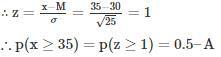= 0.5 – 0.3413 = 0.1587

*Answer can only contain numeric values
QUESTION: 9

The probability that GATE CS/IT question has an error is 1/20 and 65 questions are made in such an exam. If the probability that at least 2 question has an error is [1−[a(19/20)64]] then what is the value of (answer up to 2 decimal place)?

Solution:

Using Binomial Distribution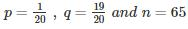Probability that x questions have an error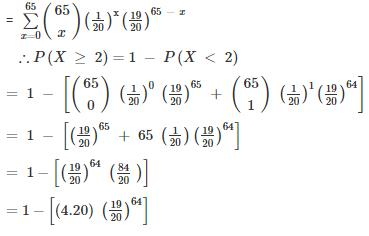QUESTION: 10

If X is a Poisson variate such that P(X=2) = 3P(X=4) then what is the value of P(X=3)?

Solution:

P (X = 2) = 3P (X = 4)